Do you like the article?
Share it with others -

Use new possibilities of MetaTrader 5

# Neural network: Self-optimizing Expert Advisor

11 October 2016, 17:24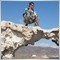3
27 695

### Introduction

After we have defined our strategy and implemented it in our Expert Advisor, we face two issues that may completely invalidate our efforts.

• What are the most suitable input values?
• How long do these values remain reliable? When do we need to perform a re-optimization?
Apart from predefined parameters (symbol, timeframe, etc.), there are other (editable) settings: indicator calculation period, buy/sell levels, TP/SL levels, etc. This may cause some issues when using the EA.

Is it possible to develop an Expert Advisor able to optimize position open and close conditions at defined intervals?

What happens if we implement a neural network (NN) in the form of a multilayer perceptron working as a module to analyze history and provide strategy? Is it possible to make the EA optimize a neural network monthly (weekly, daily or hourly) and continue its work afterwards? Thus, we arrive at the idea of ​​creating a self-optimizing EA.

This is not the first time when the topic concerning "MQL and neural network" is raised in the trading community. However, in most cases the discussion is reduced to using data obtained (sometimes manually) by an external neural network or optimizing a neural network by means of МetaTrader 4/МetaTrader 5 optimizer. Finally, EA inputs are replaced with network ones, like in this article, for instance.

In the current article, we are not going to describe a trading robot. Instead, we are going to develop and implement (module by module) a layout of an EA that is to execute the algorithm mentioned above using the multilayer perceptron (MLP) developed in MQL5 using ALGLIB library. After that, the neural network will try to solve two math tasks, the results of which can be easily checked using a different algorithm. This will help us analyze solutions and tasks that MLP plans according to its internal structure and obtain a criterion to implement an EA by simply changing the data input module. The layout should solve the self-optimization issue.

We assume that the reader is familiar with the general theory of neural networks, including their structure and organization form, number of layers, number of neurons in each layer, connections and weights, etc. In any case, the necessary information can be found in the appropriate articles.

### 1. Basic algorithm

1. Creating a neural network.
3. Normalizing data in a specific range (usually, [0, 1] or [-1, 1]).
4. Training and optimizing the neural network.
5. Calculating a network forecast and applying it according to the EA strategy.
6. Self-optimization: returning to point 2 and reiterating the process including it to the OnTimer() function.

The robot will perform regular optimization according to a user-specified time interval and the described algorithm. The user do not need to worry about it. Step 5 is not included into the reiteration process. The EA always has the forecast values even during optimization. Let's see how this happens.

### 2. ALGLIB library

The library was published and discussed in Sergey Bochkanov's publication and ALGLIB project website, http://www.alglib.net/, where it was described as a cross-platform numerical analysis and data processing library. It is compatible with a variety of programming languages (C++, C#, Pascal, VBA) and operating systems (Windows, Linux, Solaris). ALGLIB features extensive functionality. It includes:

• Linear algebra (direct algorithms, EVD/SVD)
• Linear and non-linear equation solution wizards
• Interpolation
• Optimization
• Fast Fourier transformation
• Numerical integration
• Linear and non-linear square lows
• Ordinary differential equations
• Special functions
• Statistics (descriptive statistics, hypothesis testing)
• Data analysis (classification/regression, including neural networks)
• Implementing linear algebra, interpolation and other algorithms in the high-accuracy arithmetic (using MPFR)

CAlglib class static functions are used to work with the library. This class contains all the library functions.

It features testclasses.mq5 and testinterfaces.mq5 test scripts along with a simple usealglib.mq5 demo script. The include files of the same name (testclasses.mqh and testinterfaces.mqh) are used to launch the test cases. They should be placed to \MQL5\Scripts\Alglib\Testcases\.

Out of hundreds of library files and functions, the following ones are of interest for us:

Packages
Description
alglib.mqh
The main library package includes custom functions. These functions should be called for working with the library.
dataanalysis.mqh Data analysis classes:
1. CMLPBase — multilayer perceptron.
2. CMLPTrain — training the multilayer perceptron.
3. CMLPE — sets of neural networks.

When downloading the library, the files are sent to MQL5\Include\Math\Alglib\. Include the following command to the program code to use it:

`#include <Math\Alglib\alglib.mqh>`

CAlglib class functions are applicable to the proposed solution.

```//--- create neural networks
static void    MLPCreate0(const int nin,const int nout,CMultilayerPerceptronShell &network);
static void    MLPCreate1(const int nin,int nhid,const int nout,CMultilayerPerceptronShell &network);
static void    MLPCreate2(const int nin,const int nhid1,const int nhid2,const int nout,CMultilayerPerceptronShell &network);
static void    MLPCreateR0(const int nin,const int nout,double a,const double b,CMultilayerPerceptronShell &network);
static void    MLPCreateR1(const int nin,int nhid,const int nout,const double a,const double b,CMultilayerPerceptronShell &network);
static void    MLPCreateR2(const int nin,const int nhid1,const int nhid2,const int nout,const double a,const double b,CMultilayerPerceptronShell &network);
static void    MLPCreateC0(const int nin,const int nout,CMultilayerPerceptronShell &network);
static void    MLPCreateC1(const int nin,int nhid,const int nout,CMultilayerPerceptronShell &network);
static void    MLPCreateC2(const int nin,const int nhid1,const int nhid2,const int nout,CMultilayerPerceptronShell &network)```

MLPCreate functions create a neural network with the linear output. We will create this network type in the examples in the present article.

MLPCreateR functions will create a neural network with the output within the [a, b] interval.

MLPCreateC functions will create a neural network with the output classified by "classes" (for example, 0 or 1; -1, 0 or 1).

```//--- Properties and error of the neural network
static void    MLPProperties(CMultilayerPerceptronShell &network,int &nin,int &nout,int &wcount);
static int     MLPGetLayersCount(CMultilayerPerceptronShell &network);
static int     MLPGetLayerSize(CMultilayerPerceptronShell &network,const int k);
static void    MLPGetInputScaling(CMultilayerPerceptronShell &network,const int i,double &mean,double &sigma);
static void    MLPGetOutputScaling(CMultilayerPerceptronShell &network,const int i,double &mean,double &sigma);
static void    MLPGetNeuronInfo(CMultilayerPerceptronShell &network,const int k,const int i,int &fkind,double &threshold);
static double  MLPGetWeight(CMultilayerPerceptronShell &network,const int k0,const int i0,const int k1,const int i1);
static void    MLPSetNeuronInfo(CMultilayerPerceptronShell &network,const int k,const int i,int fkind,double threshold);
static void    MLPSetWeight(CMultilayerPerceptronShell &network,const int k0,const int i0,const int k1,const int i1,const double w);
static void    MLPActivationFunction(const double net,const int k,double &f,double &df,double &d2f);
static void    MLPProcess(CMultilayerPerceptronShell &network,double &x[],double &y[]);
static double  MLPError(CMultilayerPerceptronShell &network,CMatrixDouble &xy,const int ssize);
static double  MLPRMSError(CMultilayerPerceptronShell &network,CMatrixDouble &xy,const int npoints);

```

```//--- training neural networks
static void    MLPTrainLM(CMultilayerPerceptronShell &network,CMatrixDouble &xy,const int npoints,const double decay,const int restarts,int &info,CMLPReportShell &rep);
static void    MLPTrainLBFGS(CMultilayerPerceptronShell &network,CMatrixDouble &xy,const int npoints,const double decay,const int restarts,const double wstep,int maxits,int &info,CMLPReportShell &rep);```

These functions allow you to create and optimize neural networks containing from two to four layers (input layer, output layer, zero layer, as well as one or two hidden layers). The names of the main inputs are quite self-explanatory:

• nin: number of input layer neurons.
• nout: output layer.
• nhid1: hidden layer 1.
• nhid2: hidden layer 2.
• network: CMultilayerPerceptronShell class object that is to enable definition of connections and weights between neurons and their activation function.
• xy: CMatrixDouble class object that is to enable input/output data to perform training and optimize neural networks.

Training/optimization is to be performed using the Levenberg-Marquardt (MLPTrainLM()) or L-BFGS algorithm with (MLPTrainLBFGS()) regulation. The last one is to be used if the network contains more than 500 connections/weights: function data make warnings for "networks with hundreds of weights". These algorithms are more efficient than the so-called "back propagation" commonly used in NN. The library offers other optimization functions as well. You can consider them if you have not achieved your goals using the two functions mentioned above.

### 3. Implementation in MQL

Let's define the number of neurons of each layer as external inputs. We should also define the variables containing normalization parameters.

```input int nNeuronEntra= 35;      //Number of neurons in the input layer
input int nNeuronSal= 1;         //Number of neurons in the output layer
input int nNeuronCapa1= 45;      //Number of neurons in the hidden layer 1 (cannot be <1)
input int nNeuronCapa2= 10;      //Number of neurons in the hidden layer 2 (cannot be <1)
```
```input string intervEntrada= "0;1";        //Input normalization: desired min and max (empty= NO normalization)
input string intervSalida= "";            //Output normalization: desired min and max (empty= NO normalization)
```

Other external variables:

```input int velaIniDesc= 15;
input int historialEntrena= 1500;```

They help you indicate the index of the bar (velaIniDesc), from which the history data for the network training, as well as the total bar data amount are to be uploaded (historialEntrena).

Define network object and "arDatosAprende" double array object as global open variables.
`CMultilayerPerceptronShell *objRed;`
`CMatrixDouble arDatosAprende(0, 0);`

"arDatosAprende" will include input/output data strings for the network training. This is a two-dimensional dynamic double type matrix (MQL5 allows creating only one-dimensional dynamic arrays. When creating multi-dimensional arrays, make sure to define all dimensions except the first one).

Points 1-4 of the main algorithm are implemented in the "gestionRed()" function.

```//---------------------------------- CREATE AND OPTIMIZE THE NEURAL NETWORK --------------------------------------------------
bool gestionRed(CMultilayerPerceptronShell &objRed, string simb, bool normEntrada= true , bool normSalida= true,
bool imprDatos= true, bool barajar= true)
{
double tasaAprende= 0.001;             //Network training ratio
int ciclosEntren= 2;                   //Number of training cycles
ResetLastError();
bool creada= creaRedNeuronal(objRed);                                //create the neural network
{
if(imprDatos) imprimeDatosEntra(simb, arDatosAprende);            //display data to evaluate credibility
if(barajar) barajaDatosEntra(arDatosAprende, nNeuronEntra+nNeuronSal);    //iterate over data array strings
errorMedioEntren= entrenaEvalRed(objRed, arDatosAprende, ciclosEntren, tasaAprende);      //perform training/optimization
salvaRedFich(arObjRed[codS], "copiaSegurRed_"+simb);      //save the network to the disk file
}
else infoError(GetLastError(), __FUNCTION__);

return(_LastError==0);
}
```

Here we create a NN in the function (creaRedNeuronal(objRed)); then we download data to "arDatosAprende" using the preparaDatosEntra() function. Data can be displayed for credibility evaluation using the imprimeDatosEntra() function. If input and output data should be normalized, use the normalizaDatosRed() function. Also, if you want to iterate over the data array strings before optimization, execute barajaDatosEntra(). Training is performed using entrenaEvalRed() that returns the obtained optimization error. Finally, save the network to the disk for its potential recovery without the need to create and optimize it again.

At the beginning of the gestionRed() function, there are two variables (tasaAprende and ciclosEntrena) defining NN ratio and training cycles. ALGLIB warns that they are usually used in the values the function reflects. However, while I was conducting multiple tests with the two proposed optimization algorithms, changing the values of these variables has practically no effect on the results. First, we introduced these variables as inputs, but then (because of their insignificance) I moved them inside the function. You are free to decide yourself on whether they should be treated like inputs.

normalizaDatosRed() function is to be applied to normalize NN training input data within a defined range only if real data for requesting the NN forecast are also to be located within the range. Otherwise, normalization is not necessary. Besides, real data normalization before the forecast request is not performed if the training data have already been normalized.

3.1 Creating a neural network (NN)

```//--------------------------------- CREATE THE NETWORK --------------------------------------
bool creaRedNeuronal(CMultilayerPerceptronShell &objRed)
{
int nEntradas= 0, nSalidas= 0, nPesos= 0;
if(nNeuronCapa1<1 && nNeuronCapa2<1) CAlglib::MLPCreate0(nNeuronEntra, nNeuronSal, objRed);   	//LINEAR OUTPUT
else if(nNeuronCapa2<1) CAlglib::MLPCreate1(nNeuronEntra, nNeuronCapa1, nNeuronSal, objRed);   	//LINEAR OUTPUT
else CAlglib::MLPCreate2(nNeuronEntra, nNeuronCapa1, nNeuronCapa2, nNeuronSal, objRed);   		//LINEAR OUTPUT
if(!creada) Print("Error creating a NEURAL NETWORK ==> ", __FUNCTION__, " ", _LastError);
else
{
Print("Created the network with nº layers", propiedadRed(objRed, N_CAPAS));
Print("Nº neurons in the input layer ", nEntradas);
Print("Nº neurons in the hidden layer 1 ", nNeuronCapa1);
Print("Nº neurons in the hidden layer 2 ", nNeuronCapa2);
Print("Nº neurons in the output layer ", nSalidas);
Print("Nº of the weight", nPesos);
}
}```

The above function creates NN with the necessary number of layers and neurons (nNeuronEntra, nNeuronCapa1, nNeuronCapa2, nNeuronSal) and then checks the validity of creating the network by using the function:

```//--------------------------------- EXISTING NETWORK --------------------------------------------
bool existeRed(CMultilayerPerceptronShell &objRed)
{
bool resp= false;
int nEntradas= 0, nSalidas= 0, nPesos= 0;
return(resp);
}```

If the network is set up correctly, the function informs the user about its parameters using the MLPProperties() function of the CAlglib class that can be found in ALGLIB.

As already mentioned in the section 2, ALGLIB features other functions allowing the creation of NN for classification (class label is obtained as a result) or the network for solving regression tasks (specific numeric value is obtained as a result).

After creating NN, you may define the "propiedadRed()" function to obtain some of its parameters in other EA parts:

```enum mis_PROPIEDADES_RED {N_CAPAS, N_NEURONAS, N_ENTRADAS, N_SALIDAS, N_PESOS};

//---------------------------------- NETWORK PROPERTIES  -------------------------------------------
{           //set numCapa layer index if the number of N_NEURONAS neurons is requested
int resp= 0, numEntras= 0, numSals= 0, numPesos= 0;
if(prop>N_NEURONAS) CAlglib::MLPProperties(objRed, numEntras, numSals, numPesos);
switch(prop)
{
case N_CAPAS:
resp= CAlglib::MLPGetLayersCount(objRed);
break;
case N_NEURONAS:
resp= CAlglib::MLPGetLayerSize(objRed, numCapa);
break;
resp= numEntras;
break;
case N_SALIDAS:
resp= numSals;
break;
case N_PESOS:
resp= numPesos;
}
return(resp);
}   ```

3.2  Preparing input/output data

The proposed function can change depending on the data amount and type.

```//---------------------------------- PREPARE INPUT/OUTPUT DATA --------------------------------------------------
void preparaDatosEntra(CMultilayerPerceptronShell &objRed, string simb, CMatrixDouble &arDatos, bool normEntrada= true , bool normSalida= true)
{
int fin= 0, fila= 0, colum= 0,
double valor= 0, arResp[];
arDatos.Resize(historialEntrena, nEntras+nSals);
fin= velaIniDesc+historialEntrena;
for(fila= velaIniDesc; fila<fin; fila++)
{
for(colum= 0; colum<NUM_INDIC;  colum++)
{
valor= valorIndic(codS, fila, colum);
arDatos[fila-1].Set(colum, valor);
}
calcEstrat(fila-nVelasPredic, arResp);
for(colum= 0; colum<nSals; colum++) arDatos[fila-1].Set(colum+nEntras, arResp[colum]);
}
return;
}
```

During the process, we pass along the entire history from "velaIniDesc" up to "velaIniDesc+historialEntrena" and receive the value (NUM_INDIC) of each indicator used in the strategy at each bar. After that, the values are downloaded to the appropriate columns of the CMatrixDouble two-dimensional matrix. Also, enter the strategy result ("calcEstrat()") for each bar corresponding to the specified indicator values. The "nVelasPredic" variable allows extrapolating these indicator values n candles forward. "nVelasPredic" is usually defined as an external parameter.

This means that each "arDatos" array string of the CMatrixDouble class will contain the number of columns matching the number of inputs or indicator values used in the strategy, as well as the amount of output data defined by it. "arDatos" will have the number of strings defined by the "historialEntrena" value.

3.3 Printing input/output data array

If you need to print the contents of the two-dimensional matrix to check the accuracy of input and output data, use the "imprimeDatosEntra()" function.

```//---------------------------------- DISPLAY INPUT/OUTPUT DATA --------------------------------------------------
void imprimeDatosEntra(string simb, CMatrixDouble &arDatos)
{
string encabeza= "indic1;indic2;indic3...;resultEstrat",     //indicator names separated by ";"
fichImprime= "dataEntrenaRed_"+simb+".csv";
int fila= 0, colum= 0, resultEstrat= -1, nBuff= 0,
nFilas= arDatos.Size(),
nColum= nNeuronEntra+nNeuronSal,
puntFich= FileOpen(fichImprime, FILE_WRITE|FILE_CSV|FILE_COMMON);
FileWrite(puntFich, encabeza);
for(fila= 0; fila<nFilas; fila++)
{
linea= IntegerToString(fila)+";"+TimeToString(iTime(simb, PERIOD_CURRENT, velaIniDesc+fila), TIME_MINUTES)+";";
for(colum= 0; colum<nColum;  colum++)
linea= linea+DoubleToString(arDatos[fila][colum], 8)+(colum<(nColum-1)? ";": "");
FileWrite(puntFich, linea);
}
FileFlush(puntFich);
FileClose(puntFich);
return;
}
```

The function passes along the matrix string by string creating the "línea" string at each step with all column values in the string separated by ";". These data are then passed to a .csv file created using the FileOpen() function. We will not dwell on that since this is a secondary function for the current article's subject. You can use Excel to verify .csv files.

3.4  Normalization of data within a certain interval

Usually, before we start optimizing the network, it is considered appropriate for the input data to be located within a certain range (in other words, to be normalized). To achieve this, use the following function that performs normalization of input or output data (at your choice) located in the "arDatos" array of the CMatrixDouble class:

```//------------------------------------ NORMALIZE INPUT/OUTPUT DATA-------------------------------------
{
int fila= 0, colum= 0, maxFila= arDatos.Size(),
double maxAbs= 0, minAbs= 0, maxRel= 0, minRel= 0, arMaxMinRelEntra[], arMaxMinRelSals[];
ushort valCaract= StringGetCharacter(";", 0);
if(normSalida) StringSplit(intervSalida, valCaract, arMaxMinRelSals);
{
maxAbs= arDatos[colum];
minAbs= arDatos[colum];
minRel= StringToDouble(arMaxMinRelEntra);
maxRel= StringToDouble(arMaxMinRelEntra);
for(fila= 0; fila<maxFila; fila++)                //define maxAbs and minAbs of each data column
{
if(maxAbs<arDatos[fila][colum]) maxAbs= arDatos[fila][colum];
if(minAbs>arDatos[fila][colum]) minAbs= arDatos[fila][colum];
}
for(fila= 0; fila<maxFila; fila++)                //set the new normalized value
arDatos[fila].Set(colum, normValor(arDatos[fila][colum], maxAbs, minAbs, maxRel, minRel));
}
{
maxAbs= arDatos[colum];
minAbs= arDatos[colum];
minRel= StringToDouble(arMaxMinRelSals);
maxRel= StringToDouble(arMaxMinRelSals);
for(fila= 0; fila<maxFila; fila++)
{
if(maxAbs<arDatos[fila][colum]) maxAbs= arDatos[fila][colum];
if(minAbs>arDatos[fila][colum]) minAbs= arDatos[fila][colum];
}
minAbsSalida= minAbs;
maxAbsSalida= maxAbs;
for(fila= 0; fila<maxFila; fila++)
arDatos[fila].Set(colum, normValor(arDatos[fila][colum], maxAbs, minAbs, maxRel, minRel));
}
return;
}```

Reiteration is performed if the decision is made to normalize NN training input data within a certain interval. Make sure that real data to be used for requesting the NN forecast are also inside the range. Otherwise, normalization is not required.

Remember that "intervEntrada" and "intervSalida" are string type variables defined as inputs (see the beginning of the "Implementation in MQL5" section). They may look as follows, for example "0;1" or "-1;1", i.e. contain relative highs and lows. The "StringSplit()" function passes the string to the array that is to contain these relative extreme values. The following should be done for each column:

1. Define the absolute high and low ("maxAbs" and "minAbs" variables).
2. Pass along the entire column normalizing values between "maxRel" and "minRel": see the "normValor()" function below.
3. Set a new normalized value in "arDatos" using the .set method of the CMatrixDouble class.

```//------------------------------------ NORMALIZATION FUNCTION ---------------------------------
double normValor(double valor, double maxAbs, double minAbs, double maxRel= 1, double minRel= -1)
{
double valorNorm= 0;
if(maxAbs>minAbs) valorNorm= (valor-minAbs)*(maxRel-minRel))/(maxAbs-minAbs) + minRel;
return(valorNorm);
} ```
3.5 Iteration over input/output data

In order to avoid potential value inheritance inside the data array, we can arbitrarily change (iterate) the sequence of strings inside the array. To do this, apply the "barajaDatosEntra" function that iterates over the CMatrixDouble array strings and defines a new target string for each string considering data position of each line and moving data using the bubble method ("filaTmp" variable).

```//------------------------------------ ITERATE OVER INPUT/OUTPUT DATA STRING BY STRING -----------------------------------
{
int fila= 0, colum= 0, filaDestino= 0, nFilas= arDatos.Size();
double filaTmp[];
ArrayResize(filaTmp, nColum);
MathSrand(GetTickCount());          //reset a random descendant series
while(fila<nFilas)
{
{
for(colum= 0; colum<nColum; colum++) filaTmp[colum]= arDatos[filaDestino][colum];
for(colum= 0; colum<nColum; colum++) arDatos[filaDestino].Set(colum, arDatos[fila][colum]);
for(colum= 0; colum<nColum; colum++) arDatos[fila].Set(colum, filaTmp[colum]);
fila++;
}
}
return;
}```

After resetting the random "MathSrand(GetTcikCount())" descendant series, the "randomEntero()" function becomes responsible for where exactly the strings are randomly moved to.

```//---------------------------------- RANDOM MOVING -------------------------------------------------------
int randomEntero(int minRel= 0, int maxRel= 1000)
{
int num= (int)MathRound(randomDouble((double)minRel, (double)maxRel));
return(num);
}```

3.6  Neural network training/optimization
ALGLIB library allows using the network configuration algorithms that significantly reduce training and optimization as compared to the conventional system applied in the multilayer perceptron – "back propagation". As we have already mentioned, we are to use:

• Levenberg-Marquardt algorithm with regularization and accurate (MLPTrainLM()) hessian calculation, or
• L-BFGS algorithm with (MLPTrainLBFGS()) regularization.

The second algorithm is to be used to optimize the network with the number of weights exceeding 500.

```//---------------------------------- NETWORK TRAINING-------------------------------------------
double entrenaEvalRed(CMultilayerPerceptronShell &objRed, CMatrixDouble &arDatosEntrena, int ciclosEntrena= 2, double tasaAprende= 0.001)
{
bool salir= false;
double errorMedio= 0; string mens= "Entrenamiento Red";
int k= 0, i= 0, codResp= 0,
historialEntrena= arDatosEntrena.Size();
CMLPReportShell infoEntren;
ResetLastError();
datetime tmpIni= TimeLocal();
Alert("Wait a few minutes according to the amount of applied history.");
CAlglib::MLPTrainLM(objRed, arDatosEntrena, historialEntrena, tasaAprende, ciclosEntrena, codResp, infoEntren);
else
CAlglib::MLPTrainLBFGS(objRed, arDatosEntrena, historialEntrena, tasaAprende, ciclosEntrena, 0.01, 0, codResp, infoEntren);
if(codResp==2 || codResp==6) errorMedio= CAlglib::MLPRMSError(objRed, arDatosEntrena, historialEntrena);
else Print("Cod entrena Resp: ", codResp);
datetime tmpFin= TimeLocal();
Alert("codResp ", codResp," Average training error "+DoubleToString(errorMedio, 8), " ciclosEntrena ", ciclosEntrena);
Alert("tmpEntren ", DoubleToString(((double)(tmpFin-tmpIni))/60.0, 2), " min", "---> tmpIni ", TimeToString(tmpIni, _SEG), " tmpFin ", TimeToString(tmpFin, _SEG));
infoError(GetLastError(), __FUNCTION__);
return(errorMedio);
}```

As we can see, the function receives the "network object" and the already normalized input/output data matrix as inputs. We also define cycles, or training epochs ("ciclosEntrena"; number of times the algorithm performs fitting looking for the least probable "training error"); the value recommended in the documentation is 2. Our tests did not demonstrate improved results after increasing the number of training epochs. We also mentioned the "Training ratio" ("tasaAprende") parameter.

Let's define the "infoEntren" object (of the CMLPReportShell class) at the beginning of the function that will collect training result data. After that, we will obtain it using GetNGrad() and GetNCholesky() methods. The average training error (mean square error of all output data relative to the output data obtained after processing by the algorithm) is obtained using the "MLPRMSError()" function. Besides, a user is informed on time spent for optimization. Initial and end times in tmpIni and tmpFin variables are used for that.

These optimization functions return the execution error code ("codResp") that can take the following values:

• -2 if the training sample has a number of output data exceeding the amount of neurons in the output layer.
• -1 if some function input is incorrect.
• 2 means correct execution. The error scale is less than the stop criterion ("MLPTrainLM()").
• 6 means the same for the "MLPTrainLBFGS()" function.

Thus, the correct execution will return 2 or 6 according to the number of weights of the optimized network.

These algorithms perform configuration so that reiteration of training cycles ("ciclosEntrena" variable) has almost no effect on the error obtained unlike the "back propagation" algorithm where reiteration can significantly change the obtained accuracy. The network consisting of 4 layers with 35, 45, 10 and 2 neurons and input matrix out of 2000 strings can be optimized by means of the mentioned function within 4-6 minutes (I5, core 4, RAM 8 gb) with an error of about 2-4 hundred-thousandths (4x10^-5).

3.7 Saving the network in a text file or restoring from it

At this point, we have already created NN, prepared input/output data and performed the network training. For security reasons, the network should be saved to the disk in case unexpected errors occur during the EA operation. To do this, we should use the functions provided by ALGLIB to receive the network characteristics and internal values (number of layers and neurons in each layer, value of weights, etc.) and write these data to a text file located in the disk.

```//-------------------------------- SAVE THE NETWORK TO THE DISK -------------------------------------------------
bool salvaRedFich(CMultilayerPerceptronShell &objRed, string nombArch= "")
{
int k= 0, i= 0, j= 0, numCapas= 0, arNeurCapa[], neurCapa1= 1, funcTipo= 0, puntFichRed= 9999;
double umbral= 0, peso= 0, media= 0, sigma= 0;
if(nombArch=="") nombArch= "copiaSegurRed";
nombArch= nombArch+".red";
FileDelete(nombArch, FILE_COMMON);
ResetLastError();
puntFichRed= FileOpen(nombArch, FILE_WRITE|FILE_BIN|FILE_COMMON);
{
numCapas= CAlglib::MLPGetLayersCount(objRed);
ArrayResize(arNeurCapa, numCapas);
for(k= 0; redSalvada && k<numCapas; k++)
{
arNeurCapa[k]= CAlglib::MLPGetLayerSize(objRed, k);
}
for(k= 0; redSalvada && k<numCapas; k++)
{
for(i= 0; redSalvada && i<arNeurCapa[k]; i++)
{
if(k==0)
{
CAlglib::MLPGetInputScaling(objRed, i, media, sigma);
FileWriteDouble(puntFichRed, media);
FileWriteDouble(puntFichRed, sigma);
}
else if(k==numCapas-1)
{
CAlglib::MLPGetOutputScaling(objRed, i, media, sigma);
FileWriteDouble(puntFichRed, media);
FileWriteDouble(puntFichRed, sigma);
}
CAlglib::MLPGetNeuronInfo(objRed, k, i, funcTipo, umbral);
FileWriteDouble(puntFichRed, funcTipo);
FileWriteDouble(puntFichRed, umbral);
for(j= 0; redSalvada && k<(numCapas-1) && j<arNeurCapa[k+1]; j++)
{
peso= CAlglib::MLPGetWeight(objRed, k, i, k+1, j);
}
}
}
FileClose(puntFichRed);
}
} ```

As we can see the sixth code string, .red extension is assigned to the file to simplify future searches and checks. I have spent hours debugging this function but it works!

If the work should be continued after an event that stopped the EA, we restore the network from the file on the disk using the function opposite to the one described above. This function creates the network object and fills it with data reading them from the text file where we stored the NN.

```//-------------------------------- RESTORE THE NETWORK FROM THE DISK -------------------------------------------------
bool recuperaRedFich(CMultilayerPerceptronShell &objRed, string nombArch= "")
{
bool exito= false;
int k= 0, i= 0, j= 0, nEntradas= 0, nSalidas= 0, nPesos= 0,
numCapas= 0, arNeurCapa[], funcTipo= 0, puntFichRed= 9999;
double umbral= 0, peso= 0, media= 0, sigma= 0;
if(nombArch=="") nombArch= "copiaSegurRed";
nombArch= nombArch+".red";
exito= puntFichRed!=INVALID_HANDLE;
if(exito)
{
ArrayResize(arNeurCapa, numCapas);
for(k= 0; k<numCapas; k++) arNeurCapa[k]= (int)FileReadDouble(puntFichRed);
if(numCapas==2) CAlglib::MLPCreate0(nNeuronEntra, nNeuronSal, objRed);
else if(numCapas==3) CAlglib::MLPCreate1(nNeuronEntra, nNeuronCapa1, nNeuronSal, objRed);
else if(numCapas==4) CAlglib::MLPCreate2(nNeuronEntra, nNeuronCapa1, nNeuronCapa2, nNeuronSal, objRed);
exito= existeRed(arObjRed);
if(!exito) Print("neural network generation error ==> ", __FUNCTION__, " ", _LastError);
else
{
Print("Restored the network having nº layers", propiedadRed(objRed, N_CAPAS));
Print("Nº neurons in the input layer ", nEntradas);
Print("Nº neurons in the hidden layer 1 ", nNeuronCapa1);
Print("Nº neurons in the hidden layer 2 ", nNeuronCapa2);
Print("Nº neurons in the output layer ", nSalidas);
Print("Nº of the weight", nPesos);
for(k= 0; k<numCapas; k++)
{
for(i= 0; i<arNeurCapa[k]; i++)
{
if(k==0)
{
CAlglib::MLPSetInputScaling(objRed, i, media, sigma);
}
else if(k==numCapas-1)
{
CAlglib::MLPSetOutputScaling(objRed, i, media, sigma);
}
CAlglib::MLPSetNeuronInfo(objRed, k, i, funcTipo, umbral);
for(j= 0; k<(numCapas-1) && j<arNeurCapa[k+1]; j++)
{
CAlglib::MLPSetWeight(objRed, k, i, k+1, j, peso);
}
}
}
}
}
FileClose(puntFichRed);
return(exito);
} ```

```//--------------------------------------- REQUEST THE NETWORK RESPONSE ---------------------------------
double respuestaRed(CMultilayerPerceptronShell &ObjRed, double &arEntradas[], double &arSalidas[], bool desnorm= false)
{
double resp= 0, nNeuron= 0;
if(desnorm)             //If output data normalization should be changed
{
nNeuron= ArraySize(arSalidas);
for(int k= 0; k<nNeuron; k++)
arSalidas[k]= desNormValor(arSalidas[k], maxAbsSalida, minAbsSalida, arMaxMinRelSals, arMaxMinRelSals);
}
resp= arSalidas;
return(resp);
}```

This function assumes the ability to change normalization applied to the output data in the training matrix.

### 4. Self-optimization

After the EA optimizes the neural network (as well as the inputs applied to the EA) during its operation with no optimization built into the strategy tester, the basic algorithm described in the section 1 should be repeated.

In addition, we have an important task: the EA should continuously monitor the market without losing its control during the NN optimization involving great amount of computing resources.

Let's set mis_PLAZO_OPTIM enumeration type describing the time intervals a user can select to repeat the basic algorithm (daily, selectively or at weekends). We should also set another enumeration allowing users to decide whether the EA acts as the network "optimizer" or the strategy "actuator".

```enum mis_PLAZO_OPTIM {_DIARIO, _DIA_ALTERNO, _FIN_SEMANA};
enum mis_TIPO_EAred {_OPTIMIZA, _EJECUTA};```

As you may remeber, МetaTrader 5 allows simultaneous EA execution on each open chart. Therefore, let's launch the EA in the execution mode on the first chart and run it in the optimization mode on the second one. On the first chart, the EA manages the strategy, while on the second, it only optimizes the neural networks. Thus, the second described issue is solved. On the first chart, the EA "uses" the neural network "reading" it from the text file that it generates in the "optimizer" mode each time it optimizes the NN.

We have already noted that optimization tests took about 4-6 minutes of computational time. This method slightly increases the process taking 8-15 minutes depending on Asian or European market activity time but the strategy management never stops.

In order to achieve this, we should define the following inputs.

```input mis_TIPO_EAred tipoEAred            = _OPTIMIZA;        //Executed task type
input mis_PLAZO_OPTIM plazoOptim          = _DIARIO;          //Time interval for network optimization
input int horaOptim                       = 3;                //Local time for network optimization```

"horaOptim" parameter saves the local optimization time. The time should correspond with the low or zero market activity: for example, in Europe it should be early morning (03:00 h as the default value) or on weekends. If you want to perform optimization every time the EA is launched without waiting for a defined time and day, specify as follows:

`input bool optimInicio                    = true;         //Optimize the neural network when launching the EA`

In order to define if the network is to be considered optimized ("optimizer" mode) and define the last network file reading time ("actuator" mode), we should define the following open variables:

```double fechaUltLectura;

To solve the first issue, the specified method processing block is set in the OnTimer() function that is to be executed according to "tmp" period, which in turn is set via EventSetTimer(tmp) in OnInit() at least every hour. Thus, every tmp seconds, the "optimizer" EA checks if the network should be re-optimized, while the "actuator" EA checks if the network file should be read again because it has been updated by the "optimizer".

```/---------------------------------- ON TIMER --------------------------------------
void OnTimer()
{
bool existe= false;
string fichRed= "";
if(tipoEAred==_OPTIMIZA)            //EA works in the "optimizer" mode
{
bool optimizar= false;
int codS= 0,
hora= infoFechaHora(TimeLocal(), _HORA);    //receive the full current time
fichRed= "copiaSegurRed_"+Symbol()+".red";      //define the neural network file name
existe= buscaFich(fichRed, "*.red");            //search the disk for the file where the neural network has been saved
if(!existe || optimizar)
if(hora>(horaOptim+6)) redOptimizada= false;    //upon 6 hours of the estimated time, the real optimized network is considered obsolete
}
else if(tipoEAred==_EJECUTA)        //EA works in the "actuator" mode
{
datetime fechaUltOpt= 0;
fichRed= "copiaSegurRed_"+Symbol()+".red";      //define neural network file name
existe= buscaFich(fichRed, "*.red");            //search the disk for the file where the neural network has been saved
if(existe)
{
fechaUltOpt= fechaModifFich(0, fichRed);     //define the last optimization date (network file modification)
if(fechaUltOpt>fechaUltLectura)              //if the optimization date is later than the last reading
{
recuperaRedFich(objRed, fichRed);         //read and generate the new neural network
fechaUltLectura= (double)TimeCurrent();
guardaVarGlobal(fechaUltLectura);         //save the new reading date to the disk
Print("Network restored after optimization... "+simb);      //display the message on a screen
}
}
}
return;
}```

Below are the following additional functions that are not commented upon here:

```//--------------------------------- ENABLE RE-OPTIMIZATION ---------------------------------
bool permReoptimDia()
{
int diaSemana= infoFechaHora(TimeLocal(), _DSEM);
bool permiso= (plazoOptim==_DIARIO && diaSemana!=6 && diaSemana!=0) ||     //optimize [every day from Tuesday to Saturday]
(plazoOptim==_DIA_ALTERNO && diaSemana%2==1) ||              //optimize [Tuesday, Thursday and Saturday]
(plazoOptim==_FIN_SEMANA && diaSemana==5);                   //optimize [Saturday]
return(permiso);
}

//-------------------------------------- LOOK FOR FILE --------------------------------------------
bool buscaFich(string fichBusca, string filtro= "*.*", int carpeta= FILE_COMMON)
{
bool existe= false;
string fichActual= "";
long puntBusca= FileFindFirst(filtro, fichActual, carpeta);
if(puntBusca!=INVALID_HANDLE)
{
ResetLastError();
while(!existe)
{
FileFindNext(puntBusca, fichActual);
existe= fichActual==fichBusca;
}
FileFindClose(puntBusca);
}
infoError(_LastError, __FUNCTION__);
return(existe);
```

This algorithm is currently used in the tested EA allowing us to manage the entire strategy. Every night, from 3:00 a.m. (local time) the network is re-optimized with Н1 data for 3 previous months: 35 neurons in the input layer, 45 ones in the first hidden layer, 8 in the second hidden layer and 2 in the output layer; optimization is performed 35-45 minutes.

### 5. Task 1: The binary-decimal converter

In order to check the system, we should solve the task that already has a known solution (there is an appropriate algorithm) and compare it to the one provided by the neural network. Let's create the binary-decimal converter. The following script is provided for test:

```#property script_show_confirm
#property script_show_inputs

#define FUNC_CAPA_OCULTA   1
#define FUNC_SALIDA        -5
//1= hyperbolic tangent; 2= e^(-x^2); 3= x>=0 raizC(1+x^2) x<0 e^x; 4= sigmoidal function;
//5= binomial x>0.5? 1: 0; -5= linear function
#include <Math\Alglib\alglib.mqh>

//---------------------------------  Inputs  ---------------------
sinput int nNeuronEntra= 10;                 //Number of the input layer neurons
//2^8= 256 2^9= 512; 2^10= 1024; 2^12= 4096; 2^14= 16384
sinput int nNeuronCapa1= 0;                  //Number of neurons in the first hidden layer (cannot be <1)
sinput int nNeuronCapa2= 0;                  //Number of neurons in the second hidden layer (cannot be <1)                                             //2^8= 256 2^9= 512; 2^10= 1024; 2^12= 4096; 2^14= 16384
sinput int nNeuronSal= 1;                    //Number of neurons in the output layer

sinput int    historialEntrena= 800;         //Training history
sinput int    historialEvalua= 200;          //Evaluation history
sinput int    ciclosEntrena= 2;              //Training cycles
sinput double tasaAprende= 0.001;            //Network training level
sinput string intervEntrada= "";             //Input normalization: desired min and max (empty= NO normalization)
sinput string intervSalida= "";              //Output normalization: desired min and max (empty= NO normalization)
sinput bool   imprEntrena= true;             //Display training/evaluation data

// ------------------------------ GLOBAL VARIABLES -----------------------------
int puntFichTexto= 0;
ulong contFlush= 0;
CMultilayerPerceptronShell redNeuronal;
CMatrixDouble arDatosAprende(0, 0);
CMatrixDouble arDatosEval(0, 0);
double minAbsSalida= 0, maxAbsSalida= 0;
string nombreEA= "ScriptBinDec";

//+------------------------------------------------------------------+
void OnStart()              //Binary-decimal converter
{
string mensIni= "Script conversor BINARIO-DECIMAL",
int contAciertos= 0, arNumBin[],
inicio= historialEntrena+1,
fin= historialEntrena+historialEvalua;
double arSalRed[], arNumEntra[], salida= 0, umbral= 0, peso= 0;
double errorMedioEntren= 0;
{
iniFichImprime(puntFichTexto, nombreEA+"-infRN", ".csv",mensIni);
errorMedioEntren= entrenaEvalRed(redNeuronal, arDatosAprende);
escrTexto("-------------------------", puntFichTexto);
escrTexto("RESPUESTA RED------------", puntFichTexto);
escrTexto("-------------------------", puntFichTexto);
escrTexto("numBinEntra;numDecSalidaRed;correcto", puntFichTexto);
for(int k= inicio; k<=fin; k++)
{
ArrayCopy(arNumEntra, arNumBin);
salida= respuestaRed(redNeuronal, arNumEntra, arSalRed);
salida= MathRound(salida);
correcto= k==(int)salida;
}
}
deIniFichImprime(puntFichTexto);
return;
}```

After creating the NN, we should train it with the first 800 natural numbers in binary form (10 characters, 10 input neurons and 1 output neuron). After that, we should transform the next 200 natural numbers to binary form (from 801 to 1000 in binary form) and compare the real result with the one predicted by the NN. For example, if we set 1100110100 to the network (820 in binary form; 10 characters, 10 input neurons), the network should receive 820 or some other figure close to it. The For method described above is responsible for receiving the network forecast concerning these 200 numbers and comparing the expected result with the estimated one.

After executing the script with the specified parameters (the NN without hidden layers, 10 input neurons and 1 output neuron), we obtain a great result. ScriptBinDec-infRN.csv generated in Terminal\Common\Files directory provides us with the following data: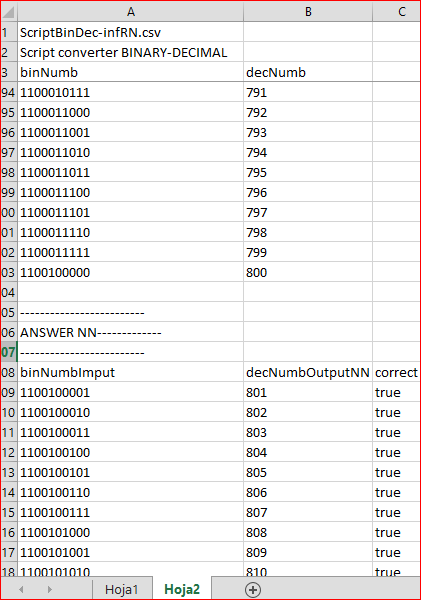As we can see, the script has printed out the training matrix up to 800 in binary (input) and decimal (output) forms. The NN has been trained and we printed out the answer starting with 801. The third column contains 'true'. This is the result of a comparison between the expected and the actual results. As already mentioned, this is a good result.

However, if we define the NN structure as "10 input neurons, 20 first hidden layer neurons, 8 second hidden layer neurons, 1 output neuron", we obtain the following: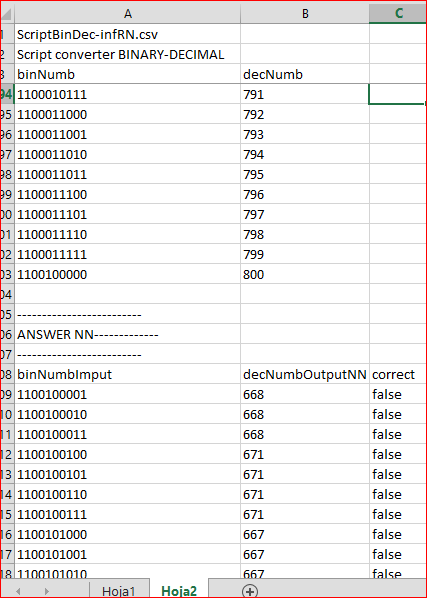This is an unacceptable result! Here we face a serious issue while processing the neural network: What is the most suitable internal configuration (number of layers, neurons and activation functions)? This issue can be solved only by solid experience, thousands of tests conducted by users and reading appropriate articles, for example "Evaluation and selection of variables for machine learning models". Besides, we applied the training matrix data in Rapid Miner statistical analysis software in order to find the most efficient structure before implementing it in MQL5.

### 6. Task 2: Prime number detector

Let's consider a similar task. This time, the NN will define whether it is a prime number or not. The training matrix will contain 10 columns with 10 characters of each natural number in binary form up to 800 and one column indicating whether it is a prime number ("1") or not ("0"). In other words, we will have 800 lines and 11 columns. Next, we should make the NN analyze the next 200 natural numbers in binary form (from 801 to 1000) and define which number is prime and which is not. Since this task is more difficult, let's print out the statistics of obtained matches.

```#include <Math\Alglib\alglib.mqh>

//---------------------------------  Inputs ----------------------- ---------------------
sinput int nNeuronEntra= 10;                 //Number of input layer neurons
//2^8= 256 2^9= 512; 2^10= 1024; 2^12= 4096; 2^14= 16384
sinput int nNeuronCapa1= 20;                 //Number of neurons in hidden layer 1 (cannot be <1)
sinput int nNeuronCapa2= 0;                  //Number of neurons in hidden layer 2 (cannot be <1)                                             //2^8= 256 2^9= 512; 2^10= 1024; 2^12= 4096; 2^14= 16384
sinput int nNeuronSal= 1;                    //Number of neurons in the output layer

sinput int    historialEntrena= 800;         //Training history
sinput int    historialEvalua= 200;          //Forecast history
sinput int    ciclosEntrena= 2;              //Training cycles
sinput double tasaAprende= 0.001;            //Network training ratio
sinput string intervEntrada= "";             //Input normalization: desired min and max (empty= NO normalization)
sinput string intervSalida= "";              //Output normalization: desired min and max (empty= NO normalization)
sinput bool   imprEntrena= true;             //Display training/evaluation data

// ------------------------------ GLOBAL VARIABLES ----------------------------------------
int puntFichTexto= 0;
ulong contFlush= 0;
CMultilayerPerceptronShell redNeuronal;
CMatrixDouble arDatosAprende(0, 0);
double minAbsSalida= 0, maxAbsSalida= 0;
string nombreEA= "ScriptNumPrimo";

//+----------------------- Prime number detector -------------------------------------------------+
void OnStart()
{
string mensIni= "Script comprobación NÚMEROS PRIMOS", cadNumBin= "", linea= "";
int contAciertos= 0, totalPrimos= 0, aciertoPrimo= 0, arNumBin[],
inicio= historialEntrena+1,
fin= historialEntrena+historialEvalua;
double arSalRed[], arNumEntra[], numPrimoRed= 0;
double errorMedioEntren= 0;
bool correcto= false,
esNumPrimo= false,
{
iniFichImprime(puntFichTexto, nombreEA+"-infRN", ".csv",mensIni);
errorMedioEntren= entrenaEvalRed(redNeuronal, arDatosAprende);
escrTexto("-------------------------", puntFichTexto);
escrTexto("RESPUESTA RED------------", puntFichTexto);
escrTexto("-------------------------", puntFichTexto);
escrTexto("numDec;numBin;numPrimo;numPrimoRed;correcto", puntFichTexto);
for(int k= inicio; k<=fin; k++)
{
esNumPrimo= esPrimo(k);
ArrayCopy(arNumEntra, arNumBin);
numPrimoRed= respuestaRed(redNeuronal, arNumEntra, arSalRed);
numPrimoRed= MathRound(numPrimoRed);
correcto= esNumPrimo==(int)numPrimoRed;
if(esNumPrimo)
{
totalPrimos++;
if(correcto) aciertoPrimo++;
}
if(correcto) contAciertos++;
escrTexto(linea, puntFichTexto);
}
}
escrTexto("porc Aciertos / total;"+DoubleToString((double)contAciertos/(double)historialEvalua*100, 2)+" %", puntFichTexto);
escrTexto("Aciertos primos;"+IntegerToString(aciertoPrimo)+";"+"total primos;"+IntegerToString(totalPrimos), puntFichTexto);
escrTexto("porc Aciertos / total primos;"+DoubleToString((double)aciertoPrimo/(double)totalPrimos*100, 2)+" %", puntFichTexto);
deIniFichImprime(puntFichTexto);
return;
}```

After executing the script with the specified parameters (the NN without hidden layers, 10 input neurons, 20 neurons in the first hidden layer and 1 in the output layer), the result is worse than the one in the previous task. ScriptNumPrimo-infRN.csv generated in Terminal\Common\Files directory provides the following data: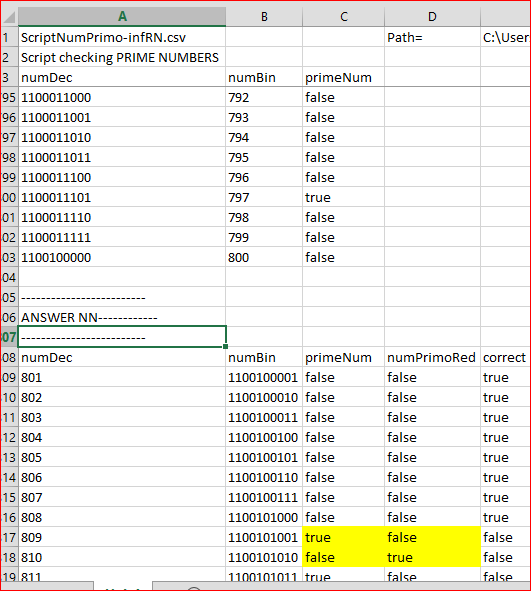Here we can see that the first prime number after 800 (809) has not been detected by the network (true = not true). Below is the statistical summary: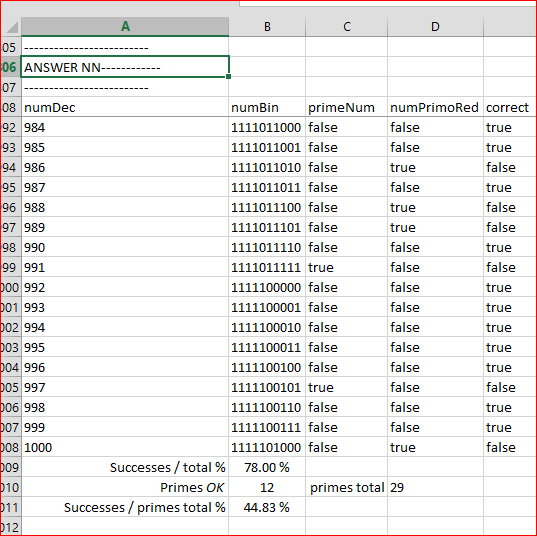The report states that the NN has managed to guess 78% of 200 numbers on the evaluation interval (801 to 200). However, out of 29 prime numbers present within the interval, it has managed to detect only 13 (44.83%).

If we conduct a test with the following network structure: "10 input layer neurons, 35 first hidden layer neurons, 10 second hidden layer neurons and 1 output layer neuron", the script displays the following data during its execution: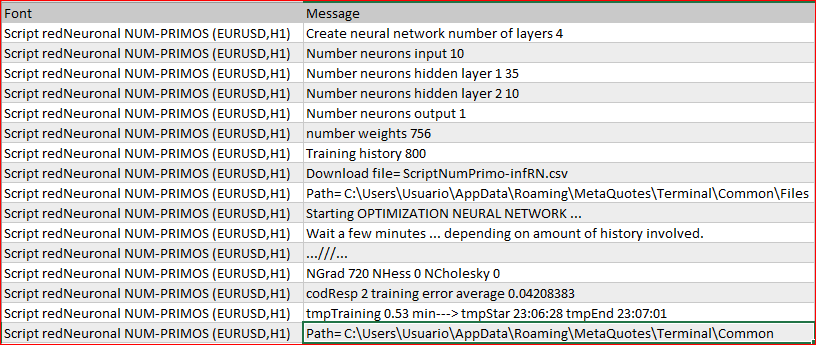As we can see on the image below, the results have worsened with the time of 0.53 minutes and average training error equal to 0.04208383.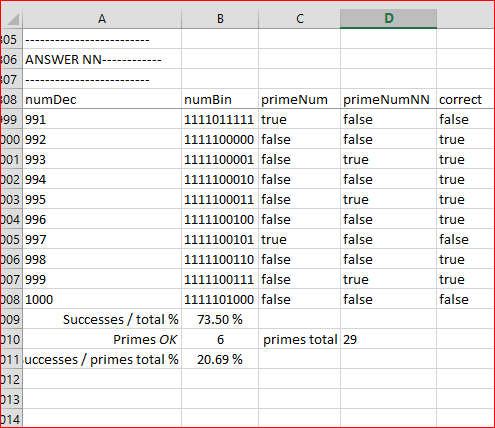Thus, we find ourselves asking again: How to define the network internal structure in the best way?

### Conclusion

While searching for a self-optimizing EA, we have implemented the neural network optimization code of ALGLIB library using the MQL5 program. We have proposed a solution for the issue that prevents the EA from managing a trading strategy when it performs the network configuration involving considerable computing resources.

After that, we used a part of the proposed code to solve two tasks from the MQL5 program: binary-decimal conversion, detecting prime numbers and tracking the results according to the NN internal structure.

Will this material provide a good basis for implementing an efficient trading strategy? We do not know yet, but we are working on it. At this stage, this article seems to be a good start.

Translated from Spanish by MetaQuotes Software Corp.
Original article: https://www.mql5.com/es/articles/2279

Last comments | Go to discussion (3)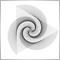| 28 Oct 2016 at 12:54

Hi and Thanks, it was great to see this self optimizer bu NN,

How can I use this in MT4? do you have the MT4 version?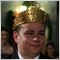| 6 Jun 2018 at 17:27

Hi, very elucidative.

Just to help, I work with AlgLib is so many platforms, and MQL is one of the most defective, like it's almost impossible to work with training tasks due to extremely poor resource management.

In this case I would use MLPSerialize, much easier to store the Network, and reliable.

Thanks anyway.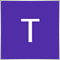| 3 Jun 2020 at 19:50

how is this an EA ? it's just a script while articles headline says

# "Neural network: Self-optimizing Expert Advisor "

i think  that its misleading since i don't see any EA attached .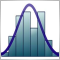Statistical Distributions in MQL5 - taking the best of R and making it faster

The functions for working with the basic statistical distributions implemented in the R language are considered. Those include the Cauchy, Weibull, normal, log-normal, logistic, exponential, uniform, gamma distributions, the central and noncentral beta, chi-squared, Fisher's F-distribution, Student's t-distribution, as well as the discrete binomial and negative binomial distributions, geometric, hypergeometric and Poisson distributions. There are functions for calculating theoretical moments of distributions, which allow to evaluate the degree of conformity of the real distribution to the modeled one.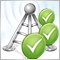The Easy Way to Evaluate a Signal: Trading Activity, Drawdown/Load and MFE/MAE Distribution Charts

Subscribers often search for an appropriate signal by analyzing the total growth on the signal provider's account, which is not a bad idea. However, it is also important to analyze potential risks of a particular trading strategy. In this article we will show a simple and efficient way to evaluate a Trading Signal based on its performance values.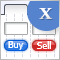Graphical Interfaces X: Updates for Easy And Fast Library (Build 3)

The next version of the Easy And Fast library (version 3) is presented in this article. Fixed certain flaws and added new features. More details further in the article.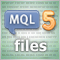MQL5 Programming Basics: Files

This practice-oriented article focuses on working with files in MQL5. It offers a number of simple tasks allowing you to grasp the basics and hone your skills.